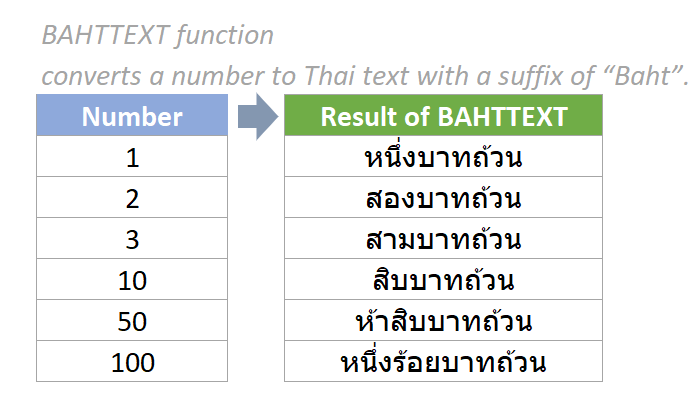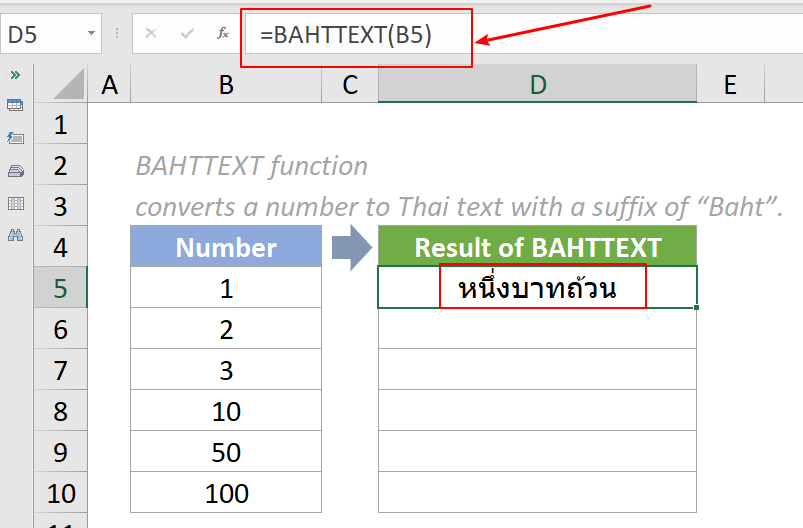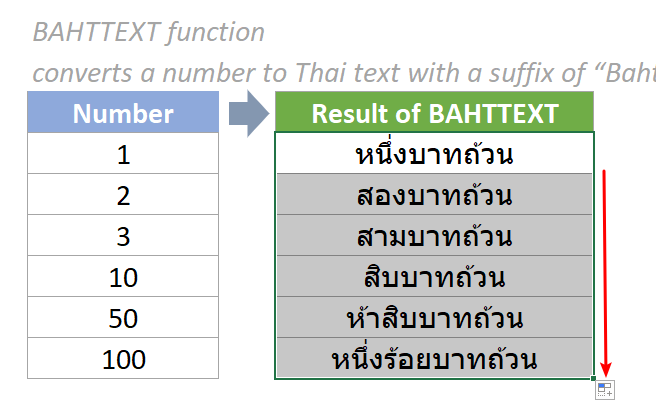## Excel BAHTTEXT Function

The BAHTTEXT Function converts a number to Thai text with a suffix of “Baht” (Thai currency บาทถ้วน).#### Syntax

=BAHTTEXT(number)

#### Arguments

• Number (required):The numeric value you want to convert to Thai text with the suffix "Baht". It can be a number, a cell reference containing a number, or a formula that evaluates to a number.

#### Return Value

The BAHTTEXT function returns a Thai text value.

#### Function notes

1. The #VALUE! error value occurs if the supplied number argument is non-numeric.

#### Example

As the below screenshot shows, there are some numbers in the range. To convert them to Thai text with "baht" appended to the end, please do as follows.

1. Please copy the formula below into cell D5, then press the Enter key to get the result.

=BAHTTEXT(B5)2. Select this result cell and drag its autofill handle down to get the rest of the results.Notes:

1. The argument in the above formula is supplied as a cell reference containing a numeric value.
2. We can also directly input a value in the formula. For example, the formula in cell D5 can be changed to:

=BAHTTEXT(1)

#### Relative Functions:

• Excel EVEN Function
The EVEN function rounds numbers away from zero to the nearest even integer.

• Excel EXP Function
The EXP function returns the result of the constant e raised to the nth power.